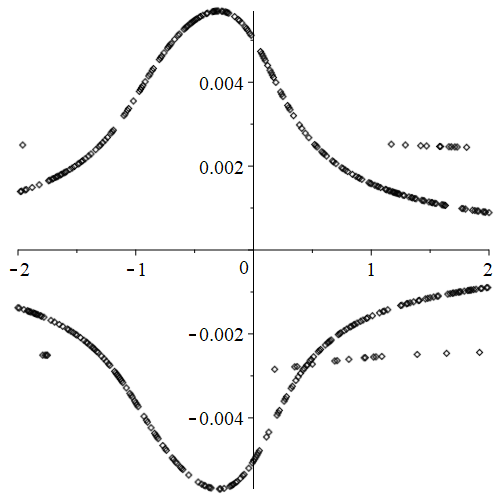## 55 Reputation

5 years, 162 days

## MaplePrimes Activity

### These are replies submitted by eager0626

 >>(1)
 >(2)
 >>Actually, i want to get the euler equation of w(Y(t),t),not just the euler equation of y(t), could  you help m?

## How to get the solution with the variabl...

Thanks for the useful scheme you proposed,

however, there are two variables of w(Y(t),t) and Y(t), it seems easy to calculate the euler equation of Y(t) through EulerLagrange or Fundiff.

I want to get the euler equation of w(Y(t),t), which failed to be solved using Fundiff as shown below:

restart;
with(Physics);
CompactDisplay(Y(t));
Y(t) will now be displayed as Y

ex := 1/2*m*(diff(Y(t), t)^2 + diff(w(Y(t), t), t)^2);
/
1   |
ex := - m |
2   \

2
/                        /[ d      ]\\
Typesetting:-mcomplete|Y[t], Typesetting:-_Hold|[--- Y(t)]||  +
\                        \[ dt     ]//

/
|D(w)(Typesetting:-mcomplete(Y, Typesetting:-_Hold([Y(t)])),
\

/                        /[ d      ]\\
t) Typesetting:-mcomplete|Y[t], Typesetting:-_Hold|[--- Y(t)]|| +
\                        \[ dt     ]//

D(w)(Typesetting:-mcomplete(Y, Typesetting:-_Hold([Y(t)])), t

\
\  |
)|^2|
/  /

RR := Intc(ex, t);
/infinity     /
|          1   |
RR :=  |          - m |
|          2   \
/-infinity

2
/                        /[ d      ]\\
Typesetting:-mcomplete|Y[t], Typesetting:-_Hold|[--- Y(t)]||  +
\                        \[ dt     ]//

/
|D(w)(Typesetting:-mcomplete(Y, Typesetting:-_Hold([Y(t)])),
\

/                        /[ d      ]\\
t) Typesetting:-mcomplete|Y[t], Typesetting:-_Hold|[--- Y(t)]|| +
\                        \[ dt     ]//

D(w)(Typesetting:-mcomplete(Y, Typesetting:-_Hold([Y(t)])), t

\
\  |
)|^2| dt
/  /

Fundiff(RR, Y);
/
|
-|D(w)(Typesetting:-mcomplete(Y, Typesetting:-_Hold([Y(t)])), t
\

/
)^2 Typesetting:-mcomplete|Y[tcommat],
\

/
/[ d  / d      \]\\   |
Typesetting:-_Hold|[--- |--- Y(t)|]|| + |
\[ dt \ dt     /]//   \

2
/                        /[ d      ]\\
Typesetting:-mcomplete|Y[t], Typesetting:-_Hold|[--- Y(t)]||
\                        \[ dt     ]//

D[1, 1](w)(

Typesetting:-mcomplete(Y, Typesetting:-_Hold([Y(t)])), t) + 2

D[1, 2](w)(

Typesetting:-mcomplete(Y, Typesetting:-_Hold([Y(t)])), t)

/                        /[ d      ]\\
Typesetting:-mcomplete|Y[t], Typesetting:-_Hold|[--- Y(t)]|| +
\                        \[ dt     ]//

D[2, 2](w)(

\
|
Typesetting:-mcomplete(Y, Typesetting:-_Hold([Y(t)])), t)|
/

D(w)(Typesetting:-mcomplete(Y, Typesetting:-_Hold([Y(t)])), t

/
) + Typesetting:-mcomplete|Y[tcommat],
\

\
/[ d  / d      \]\\|
Typesetting:-_Hold|[--- |--- Y(t)|]||| m
\[ dt \ dt     /]///

Fundiff(RR, w(Y(t), t));
Error, (in Physics:-Fundiff) all the arguments of the differentiation function w(Y(t), t) must be different from those used for w inside (1/2)*m*((diff(Y(t), t))^2+((D(w))(Y(t), t)*(diff(Y(t), t))+(D(w))(Y(t), t))^2)

## fsolve...

As you have mentioned, the comman "fsolve" may be the efficient scheme to solve this multi-value problem compared to the

method using DirectSearch and Basis.

Thanks!

## Time consuming...

@vv Thanks for your professional and comprehensive reply of the solution finding method using Basis command.

It is very useful for me in root finding proceedings of a complex equations.

However, it seems a relative long time consumption is necessary in solving procedure using Basis which is not benifit to be adopted in a parametric study of sigma.

I am looking for a efficient method to solve this kind of equation.

Thanks again!

## Solution finding...

I have realized the infinity solution problem in the equations as you mentioned.

However, I can get some solutions when using fsolve as changing the parameter sigma even though i don't know if it correct. it can be seen that there are some solution missed.Following the hint you have given, i replaced the sin and cos with c and d. And I also tried to use the Groebner:-Basis, but i couldn't get any solution.

Could you give me some hint how to solve it assuming Upsilon to be bounded in a certain range.

nonlinearsystemforallsolution_1.mw

## Trival solution...

Actually, Based on the resultes calculated by the "DirectSearch package", it seems just trival solution (near zero) is found.

However, there exist the non-trival solution calculated by the "fsolve" , it has been showed as [0.000454, -0.0027,-27.316].

## @Kitonum  Thank you very much to le...

@Kitonum
Thank you very much to let me know this useful command.

It becomes clear to understand to convert it to this form.

Thanks!

## @Carl Love  Thank you very much fo...

There is some error in my proposed solution.

And, I understood the solution from Maple through your explanation.

Thanks!